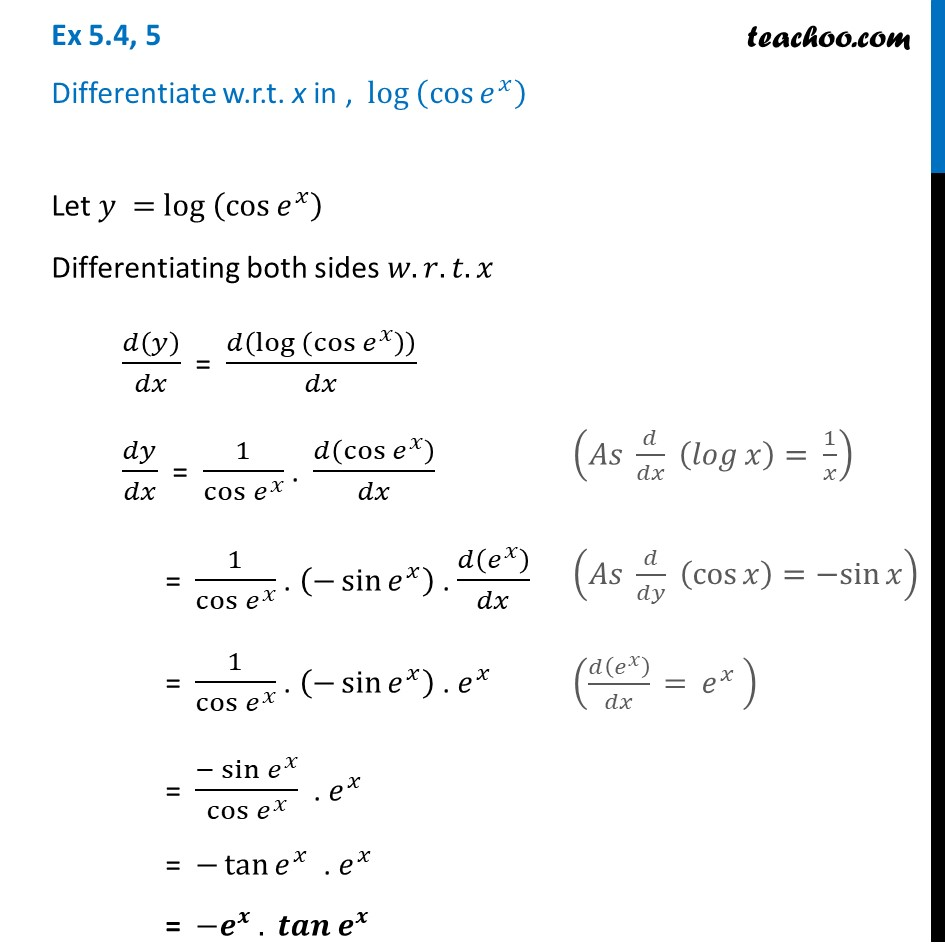Finding derivative of Exponential & logarithm functions

Chapter 5 Class 12 Continuity and Differentiability
Concept wiseLearn in your speed, with individual attention - Teachoo Maths 1-on-1 Class

### Transcript

Ex 5.4, 5 Differentiate w.r.t. x in , 〖log 〗⁡(cos⁡〖𝑒^𝑥 〗 )Let 𝑦 = 〖log 〗⁡(cos⁡〖𝑒^𝑥 〗 ) Differentiating both sides 𝑤.𝑟.𝑡.𝑥 𝑑(𝑦)/𝑑𝑥 = 𝑑(〖log 〗⁡(cos⁡〖𝑒^𝑥 〗 ) )/𝑑𝑥 𝑑𝑦/𝑑𝑥 = 1/cos⁡〖𝑒^𝑥 〗 . 𝑑(cos⁡〖𝑒^𝑥 〗 )/𝑑𝑥 = 1/cos⁡〖𝑒^𝑥 〗 . (−sin⁡〖𝑒^𝑥 〗 ) . 𝑑(𝑒^𝑥 )/𝑑𝑥 = 1/cos⁡〖𝑒^𝑥 〗 . (−sin⁡〖𝑒^𝑥 〗 ) . 𝑒^𝑥 = (−sin⁡〖𝑒^𝑥 〗)/cos⁡〖𝑒^𝑥 〗 . 𝑒^𝑥 = −tan⁡〖𝑒^𝑥 〗 . 𝑒^𝑥 = −𝒆^𝒙 . 𝒕𝒂𝒏⁡〖𝒆^𝒙 〗 (𝐴𝑠 𝑑/𝑑𝑥 (𝑙𝑜𝑔⁡𝑥 )= 1/𝑥) (𝐴𝑠 𝑑/𝑑𝑦 (cos⁡𝑥 )=〖−sin〗⁡𝑥 )